# Monocular slam 中的理论基础(2)

## 三角法求深度（triangulation）

在知道了相机的轨迹以后，使用三角法就能计算某个点的深度，在Hartley的《Multiple view Geometry》一书中第10章、第12章都是讲的这个，这里只讲解线性求解方法。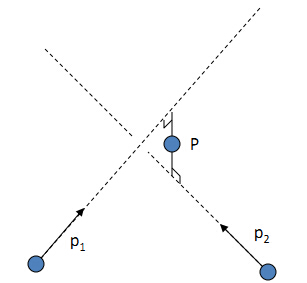对于三维空间中的一点 P $P$，我们假设第一个摄像机坐标系C1$C_{1}$就是世界坐标系，P在世界坐标系下的表示为 P=(x,y,z,1)T $P=(x,y,z,1)^T$，这时，摄像机坐标系 C1 $C_{1}$的外参数矩阵 M1 $M_{1}$为单位矩阵。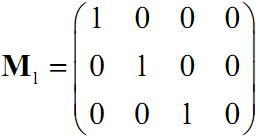P $P$和光心的连线交第一个图像平面于点p1$p_{1}$ ,注意这里的 p1 $p_{1}$是在摄像机坐标系的坐标表示，不是在图像坐标系下，这在上一篇博客中已经强调。同理，和第二个摄像机光心连线交第二幅图像于点 p2 $p_{2}$ pi $p_{i}$在各自摄像机坐标系中的表示为：
p1=x1y11c1 $p_{1} =\begin{pmatrix} x_{1}\\ y_{1}\\ 1 \end{pmatrix}_{c_{1}}$  和  p2=x2y21c2 $p_{2} =\begin{pmatrix} x_{2}\\ y_{2}\\ 1 \end{pmatrix}_{c_{2}}$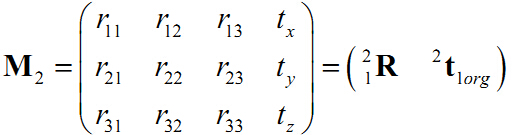12H=[R3×30t3×11] $_{2}^{1}H = \begin{bmatrix} R_{3\times3} & t_{3\times1}\\ 0& 1\end{bmatrix}$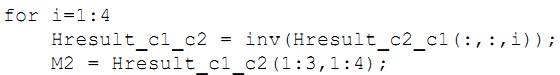有了外参矩阵，我们就可以得到这些点坐标的关系：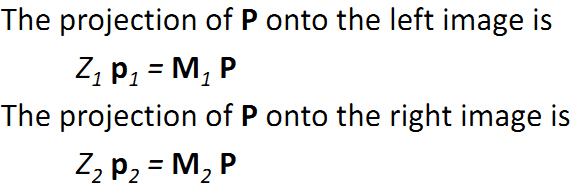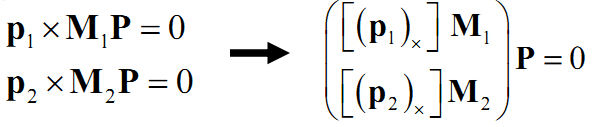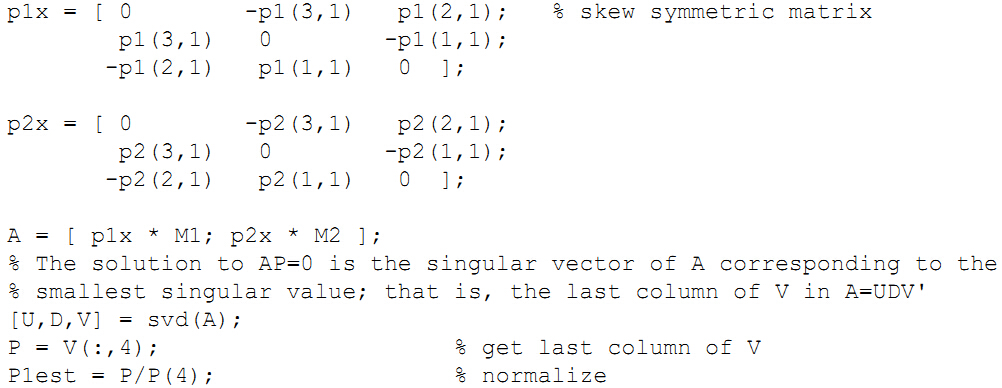计算出来的P的坐标就是P在世界坐标系中的坐标，这里就是P在摄像机坐标系 C1 $C_{1}$中的表示。并且注意上篇博客中强调过的：
p1=K1u1v11 $p_1=K^{-1}\begin{pmatrix} u_{1}\\ v_{1}\\ 1 \end{pmatrix}$ p2=K1u2v21 $p_2=K^{-1}\begin{pmatrix} u_{2}\\ v_{2}\\ 1 \end{pmatrix}$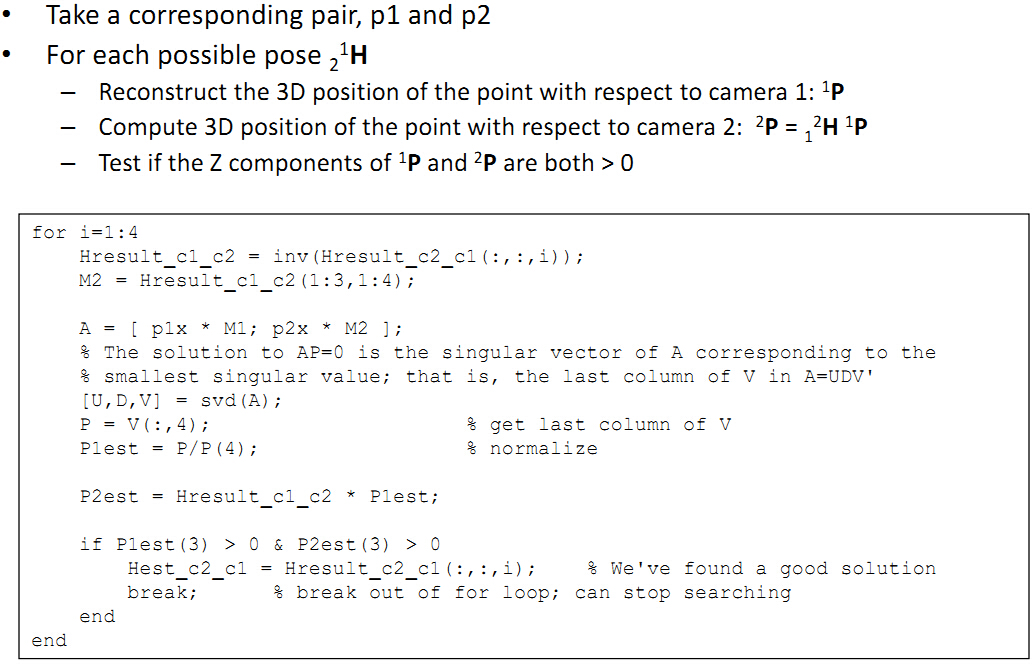在以上计算P三维坐标的推导过程中，可以看到和本征矩阵E是息息相关的，E和我们的尺度紧密相连，所以计算出来的深度和尺度scale也是直接相关的。同时，根据这种三角法(triangulation )计算的深度，其实是不怎么靠谱的，一般只是拿这个做一个初始值。并且，我们还可以初略的看看深度估计误差和什么有关。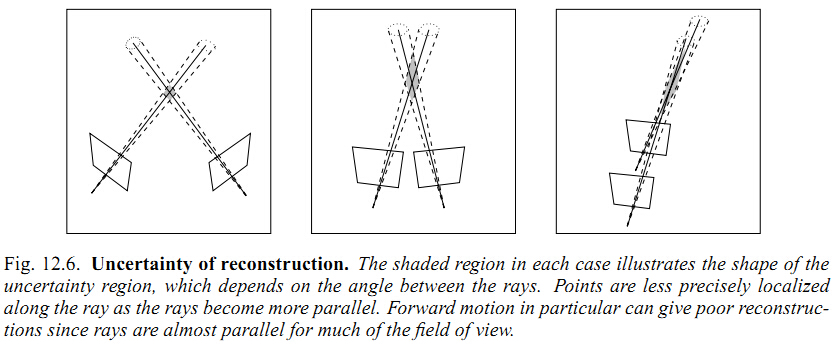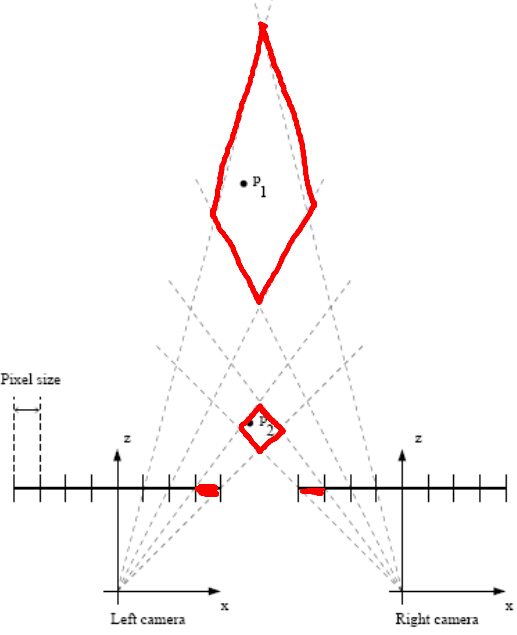在理顺了这系列流程，有了基本的视觉基础以后，就可以开始向svo，orb_slam，lsd_slam前进了。但是这些算法的深度估计都是用概率模型来更新深度，不用怕，不变应万变，在后续的博客中，我们将一一剖析。最后，再推荐下这本书《Mastering OpenCV with Practical Computer Vision Projects》，里面有一章专门教一步步用opencv写structure from motion的程序，内容实在是牛的飞，赶紧去看看动手自己写写程序吧，祝好。

（转载请注明作者和出处： http://blog.csdn.net/heyijia0327 未经允许请勿用于商业用途）

reference：10-124万+
06-22295
07-055555
10-154万+
01-115040
09-212025
10-123万+
08-067518